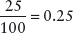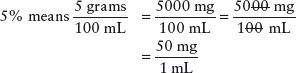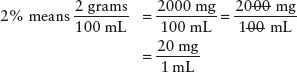# Mathematics and Calculations for the Veterinary Anesthetist

1. Right Patient

2. Right Agent

3. Right Dose

4. Right Route

5. Right Time

6. Right Technique

7. Right Documentation

# The Basics: Metric System

Conversion of units requires the use of a “conversion factor,” which is any equivalent equation in which the known and the unknown units are involved (Tables 3.13.4) (Bill 2000). You must set up a proportion of values on either side of the equation.Cross-multiply and divide.50 pounds = 22.7 kg

Table 3.1. Weight.

 Unit Fraction Symbol Kilogram 1000 grams kg Gram 1000 milligrams g Milligram 1/1000 of a gram mg Microgram 1/1,000,000 of a gram μcg or μg

Table 3.2. Weight.Table 3.3. Volume.Table 3.4. Common unit conversions.

 1 kilogram = 2.2 pounds 1 teaspoon = 5 mL 1 tablespoon = 15 mL 1 grain = 60 mg

# Percentages

Percent can be expressed as “out of 100” or “for every 100.” For example: 25% can be written asThe decimal point can be moved two places to the left to achieve the same calculation.## Agent strength as percent solution

Agents with the dose listed as a concentration percent represent grams in 100 mL.

Lasix as a 5% solution has a concentration of 50 mg/mL.Lidocaine as a 2% solution has a concentration of 20 mg/mL.The decimal point can be moved one place to the right in any percent solution to discern the mg/mL concentration.